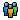问答管理员：传输

LTE信道估计

sinc的矩形窗是用在频域吧？你查查sinc滤波器的作用，其实就是时域信号生成的时候就要用sinc函数生成，这样保证信号在频率是有限范围（不然无限带宽也发不出去啊）。可以参考matlab的sinc例子。rng default

t = 1:10;
x = randn(size(t))';
ts = linspace(-5,15,600);
[Ts,T] = ndgrid(ts,t);
y = sinc(Ts - T)*x;

plot(t,x,'o',ts,y)
xlabel Time, ylabel Signal
legend('Sampled','Interpolated','Location','SouthWest')
legend boxoff
figure;plot(abs(fftshift(fft(y))));

 我要回答： 回答字数在10000字以内